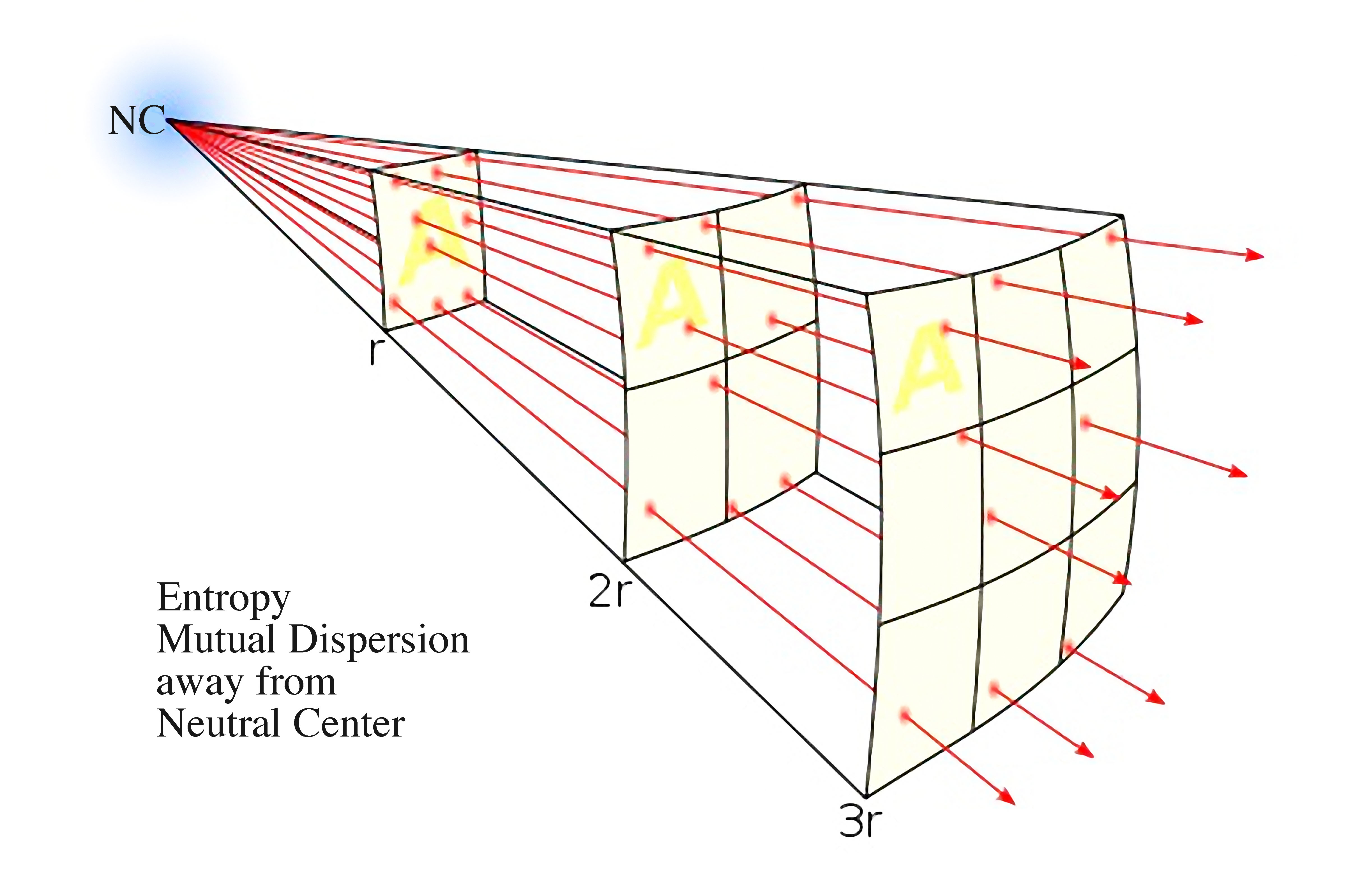Loading...

# Inverse Square Law

Inverse Square Law: The inverse-square law, in physics, is any physical law stating that a specified physical quantity or intensity is inversely proportional to the square of the distance from the source of that physical quantity. The fundamental cause for this can be understood as geometric dilution corresponding to point-source radiation into three-dimensional space." Wikipedia, Inverse Square Law(click to enlarge)

Reciprocal of Square Law. See Syntropy

See Also

Ellipse
exponentiation
Chart of Locked Potentials
Fibonacci
Fibonacci Relationships
Fibonacci Series
Locked Potentials and the Square Law
Part 12 - Russells Locked Potentials
Quantum Arithmetic Elements
Scale of Locked Potentials
Square Law
3.04 - Power Accumulation via Fibonacci-like Patterns
Page last modified on Thursday 22 of March, 2018 06:44:07 MDT